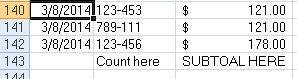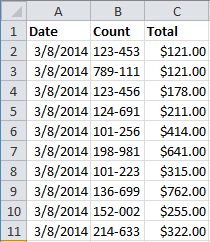# is this possible - excel insert totals

Go to solution Solved by JLogan3o13,

## Recommended Posts

I have an excel file that contains an account number and a dollar amount. Each day the file will have a different number of rows. Is it possible to have AutoIt open the excel file, determine the last row, and insert a count on the account number field and a sum on the dollar amount field?

##### Share on other sites

• Moderators

It is possible. If you want to write on the last row with data in it, you could do this:

```Const \$xlCellTypeLastCell = 11

\$oExcel = ObjCreate("Excel.Application")
\$oBook = \$oExcel.Workbooks.Open("C:\Users\JeremiaL\Desktop\Test.xls")
\$oExcel.Visible = True
\$oSheet = \$oBook.Worksheets(1)
\$oSheet.Activate

\$oRange = \$oSheet.UsedRange
\$oRange.SpecialCells(\$xlCellTypeLastCell).Activate```

If you want the first open row, add this to the end:

```\$newRow = \$oExcel.ActiveCell.Row + 1
\$oExcel.Range("A" & \$newRow).Activate```

"Profanity is the last vestige of the feeble mind. For the man who cannot express himself forcibly through intellect must do so through shock and awe" - Spencer W. Kimball

##### Share on other sites

It is possible. If you want to write on the last row with data in it, you could do this:

```Const \$xlCellTypeLastCell = 11

\$oExcel = ObjCreate("Excel.Application")
\$oBook = \$oExcel.Workbooks.Open("C:\Users\JeremiaL\Desktop\Test.xls")
\$oExcel.Visible = True
\$oSheet = \$oBook.Worksheets(1)
\$oSheet.Activate

\$oRange = \$oSheet.UsedRange
\$oRange.SpecialCells(\$xlCellTypeLastCell).Activate```

If you want the first open row, add this to the end:

```\$newRow = \$oExcel.ActiveCell.Row + 1
\$oExcel.Range("A" & \$newRow).Activate```

Thanks, that gets me part of the way there. Any idea how to get it to insert a 'sum' and a 'count'?

##### Share on other sites

• Moderators

So you want a sum of all dollar amounts? How about a screenshot of an excel example, so we can see how you have/want the data laid out? Then it should be relatively easy to get you where you want to be.

"Profanity is the last vestige of the feeble mind. For the man who cannot express himself forcibly through intellect must do so through shock and awe" - Spencer W. Kimball

##### Share on other sites

So you want a sum of all dollar amounts? How about a screenshot of an excel example, so we can see how you have/want the data laid out? Then it should be relatively easy to get you where you want to be.

screenshot attached...Edited by erik7426
##### Share on other sites

• Moderators
• Solution

Ok, I'm not sure what the purpose of the count numbers are, or how you're totaling them, but for a subtotal you could do something like this:

Beginning with this:You could do something like this:

```#include <Excel.au3>

Local \$subTotal, \$grandTotal
\$oExcel = _ExcelBookOpen(@DesktopDir & "\Test.xls")
\$oRange = \$oExcel.Worksheets(1).UsedRange
\$oRange.SpecialCells(\$xlCellTypeLastCell).Activate
\$oRow = \$oExcel.ActiveCell.Row
\$subTotalArray = _ExcelReadArray(\$oExcel, 2, 3, \$oRow, 1)

For \$element in \$subTotalArray
\$subTotal += \$element
Next

\$grandTotal = _ExcelWriteCell(\$oExcel, \$subTotal, \$oRow + 1, 3)```

"Profanity is the last vestige of the feeble mind. For the man who cannot express himself forcibly through intellect must do so through shock and awe" - Spencer W. Kimball

## Create an account

Register a new account

• ### Recently Browsing   0 members

×

• Wiki

• Back

• #### Beta

• Git
• FAQ
×
• Create New...